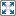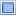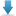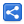[Last Updated: Sep 25, 2018]

Function overloading is a feature which allows to create multiple functions with same name but with different parameters.

TypeScript provides a way to create overloaded functions which allows us to provide a list of functions without implementations. We need to provide the implementation as a combination of all listed functions.

# Examples

```//overloaded functions
function sum(x: number, y: number): number;
function sum(x: number, y: number, z: number): number;
//the combined implementation
function sum(x: number, y: number, z?: number): number {
if (typeof z == 'undefined') {
return x + y;
} else {
return x + y + z;
}
}

let n = sum(1, 2);//calling first overloaded function
console.log(n);
n = sum(1, 2, 3);//calling second overloaded function
console.log(n);```

#### Output

`36`

The corresponding compiled JavaScript code:

```function sum(x, y, z) {
if (typeof z == 'undefined') {
return x + y;
}
else {
return x + y + z;
}
}
let n = sum(1, 2);
console.log(n);
n = sum(1, 2, 3);
console.log(n);
```

Following is another example which uses different return types.

```function divide(x: number, y: number): number;
function divide(str: string, y: number): string[];

function divide(x: any, y: number): any {
if (typeof x == 'number') {
return x / y;
} else if (typeof x == 'string') {
return [x.substring(0,y), x.substring(y)];
}
}

let n: number = divide(6, 2);
console.log(n);
let s: string[] = divide("football",4);
console.log(s);```

#### Output

`3[ 'foot', 'ball' ]`

The corresponding compiled JavaScript code:

```function divide(x, y) {
if (typeof x == 'number') {
return x / y;
}
else if (typeof x == 'string') {
return [x.substring(0, y), x.substring(y)];
}
}
let n = divide(6, 2);
console.log(n);
let s = divide("football", 4);
console.log(s);
```

Similarly a class can also have overloaded static/member methods:

```class Util {
static divide(x: number, y: number): number;
static divide(str: string, y: number): string[];
static divide(x: any, y: number): any {
if (typeof x == 'number') {
return x / y;
} else if (typeof x == 'string') {
return [x.substring(0, y), x.substring(y)];
}
}
}

let n: number = Util.divide(6, 2);
console.log(n);
let s: string[] = Util.divide("football", 4);
console.log(s);```

#### Output

`3[ 'foot', 'ball' ]`

## Example Project

Dependencies and Technologies Used:

• TypeScript 3.0.3
 Function Overloading in TypeScriptSelect AllDownloadShare